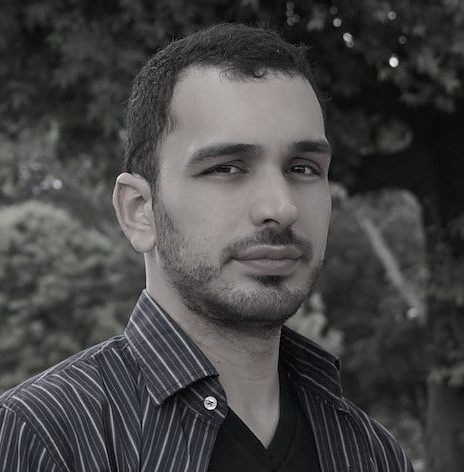Visiting Speaker
###### Bruno Ribeiro
Assistant Professor of Computer Science, Purdue University
New Directions in Representation Learning for Graphs
Monday
Oct 7, 2019
Watch video
10:30 am
177 Huntington Ave
11th floor

My talk starts by turning back the clock to 1931 and 1981, introducing the ideas that culminated with a fundamental representation theorem of graphs (the Aldous–Hoover theorem). I will then show how these ideas connect to a probabilistic interpretation of matrix factorization methods, explaining why matrix factorization is fundamentally not as expressive as it could be to describe finite graphs. I will then introduce the concept of representation learning with graph neural networks (GNNs) and explain its connections to statistical graph models and the Weisfeiler-Lehman isomorphism test. Finally, I will introduce a newly proposed general framework for graph representation learning using deep neural networks, which is directly rooted in the ideas that gave us the Aldous–Hoover representation theorem. This new representation framework points to novel graph models, new approaches to make existing graph model more scalable, and provides a unifying approach connecting matrix factorization, graph mining algorithms, and graph neural networks. I will end my talk with a few open problems.

This is joint work with Ryan Murphy, Bala Shrinivasan, and Vinayak Rao.

Bruno Ribeiro is an Assistant Professor in the Department of Computer Science at Purdue University. He obtained his Ph.D. at the University of Massachusetts Amherst and did his postdoctoral studies at Carnegie Mellon University from 2013-2015. His research interests are in deep learning and data mining, with a focus on sampling and modeling relational and temporal data.
Visiting Speaker###### Bruno Ribeiro
Assistant Professor of Computer Science, Purdue University
New Directions in Representation Learning for Graphs
Mon
Oct 7, 2019
10:30 am
177 Huntington Ave
11th floor

My talk starts by turning back the clock to 1931 and 1981, introducing the ideas that culminated with a fundamental representation theorem of graphs (the Aldous–Hoover theorem). I will then show how these ideas connect to a probabilistic interpretation of matrix factorization methods, explaining why matrix factorization is fundamentally not as expressive as it could be to describe finite graphs. I will then introduce the concept of representation learning with graph neural networks (GNNs) and explain its connections to statistical graph models and the Weisfeiler-Lehman isomorphism test. Finally, I will introduce a newly proposed general framework for graph representation learning using deep neural networks, which is directly rooted in the ideas that gave us the Aldous–Hoover representation theorem. This new representation framework points to novel graph models, new approaches to make existing graph model more scalable, and provides a unifying approach connecting matrix factorization, graph mining algorithms, and graph neural networks. I will end my talk with a few open problems.

This is joint work with Ryan Murphy, Bala Shrinivasan, and Vinayak Rao.